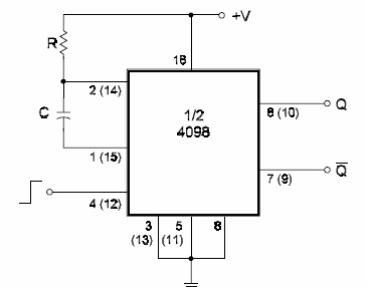DatasheetsN° of component

Monostable Using the 4098 (I) (CB137)

The 4098 IC contains two monostable multivibrators in the same package. The basic configuration for this IC is shown here. The numbers in brackets represent the pins of the second multivibrator. This is a retriggerable and re-setable multivibrator triggered by the positive transition of the input signal. The minimum value for R is 5 k ohm and the maximum capacitance is 100 µF The time constant is 0.5 x R x C. The circuit has complementary outputs.Monostable Using the 4098 (I)

Datasheet of 4098